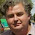## Friday, November 6, 2015

### Two new connections between the Planetary and Lunar Cycles

updated: 09/11/2015 (see bottom of post)

1. The Connection Between the Lunar Tidal Cycles and the Synodic Period of Venus and the Earth.
The first direct connection between the planetary orbital periods and the lunar tidal cycles can be found in a previous blog post that is located at:
In this post it was found that:
If you take the minimum period between the times of maximum change in the tidal stresses acting upon the Earth that are caused by changes in the direction of the lunar tides (i.e. 1.89803 tropical years), and amplitude modulate this period by the minimum period between the times of maximum change in tidal stresses acting upon the Earth that are caused by changes in the strength of the lunar tides (i.e. 10.14686 tropical years), you find that the 1.89803 year tidal forcing term is split into a positive and a negative side-lobe, such that:
Positive side-lobe
[10.14686 x 1.89803] / [10.14686 – 1.89803] = 2.3348 tropical yrs = 28.02 months

Negative side-lobe
[10.14686 x 1.89803] / [10.14686 + 1.89803] = 1.5989 tropical yrs

N.B.
10.146856 tropical years = 3706.059873 days = 9.0 Full Moon Cycles (FMC) and
1.0 FMC = 411.78443029 days = 1.127428 tropical years.
1.89803 tropical years = 693.2401518 days = 2.0 Draconic Years and
1.0 Draconic Year = 346.62007588 days = 0.949014 tropical years.
The time period of the positive side-lobe is almost exactly the same as that of the Quasi-Biennial Oscillation (QBO). The QBO is a quasi-periodic oscillation in the equatorial stratospheric zonal winds that has a mean period of oscillation of approximately 28 months.
Even more remarkable is the time period of the negative side-lobe. It is almost exactly the same as that of the synodic period of the orbits of Venus and the Earth (i.e. 583.92063 days = 1.5987 tropical years), agreeing to within an error of only ~ 1.8 hours.

2. The Connection Between the Lunar Tidal Cycles and the Synodic Period of Jupiter and Saturn.
The second  direct connection between the planetary orbital periods and the lunar tidal cycles comes from a relationship that links the period of the QBO to the lunar and planetary cycles.

(8/19.8592) + (8/18.6000) + (4/8.8505) = 3/(2.3348)

where

19.8592 tropical yrs = the synodic period of Jupiter and Saturn.
18.6000 tropical yrs = time for the lunar line-of-nodes to precess around the Earth w.r.t. the stars.
8.8505 tropical yrs = time for the lunar line-of-apse to precess around the Earth w.r.t. the stars.
2.3348 tropical yrs = 28.02 months approximately equal to the average length of the QBO.

This can be rewritten as:

(8/19.8592) + (8/9.0697) = 3/(2.3348)

Where 9.0697 tropical years is half the harmonic mean of 17.7010 ( = 2 x 8.8505) tropical years and 18.6000 tropical years. This is close to the 9.1 tropical year spectral peak that is known as the the quasi-decadal oscillation.

Hence, we have an expression where the first term on the left is a bi-decadal oscillation, the second term on the left is a quasi-decadal oscillation and the denominator of the first term on the right is near to, but not precisely at, the nominal QBO oscillation period of 2.371 tropical years.

Remarkably, however, the  denominator of the first term on the right-hand side is exactly that of the positive side-lobe produced by the amplitude modulation in part 1.

Hnece, we have established two new connections between the synodic periods of Earth/Venus and Jupiter/Saturn that directly link into the variations in the stresses placed upon the Earth's atmosphere and oceans by the luni-solar tidal cycles

UPDATE: 09/11/15

A third connection between the orbital period of Jupiter and the lunar Draconic year.

In my 2008 paper (that was eventually published in 2010) i.e.

Wilson, I.R.G., 2011, Are Changes in the Earth’s Rotation
Rate Externally Driven and Do They Affect Climate?
The General Science Journal, Dec 2011, 3811.

http://gsjournal.net/Science-Journals/Essays/View/3811

I showed that:

5.0 Draconic years = 0.4 Jupiter orbits = 4 x 433.275 days = 4 x Chandler wobble = 2 x QBO

(with 1 Jupiter orbit = 4332.75 days = 11.8627 tropical years)

This is equivalent to:

50 Draconic years = 4 x 11.8627 topical years = 4 x orbital period of Jupiter.

Thanks oldbrew for reminding every one of this additional connection between the Lunar Draconic cycle and orbit of Jupiter that I made back in 2008.

1.This is some really nice work Ian!

2.Thanks for the complement Tom! I have discovered three directconnections between the orbital periods of Venus, the Earth and Jupiter and the lunar tidal cycles.

3.I am very interested in the 3.79 year cycle.
Is this related to atmospheric pressure? Or is it a wave cycle?
Are there any papers written on this cycle?